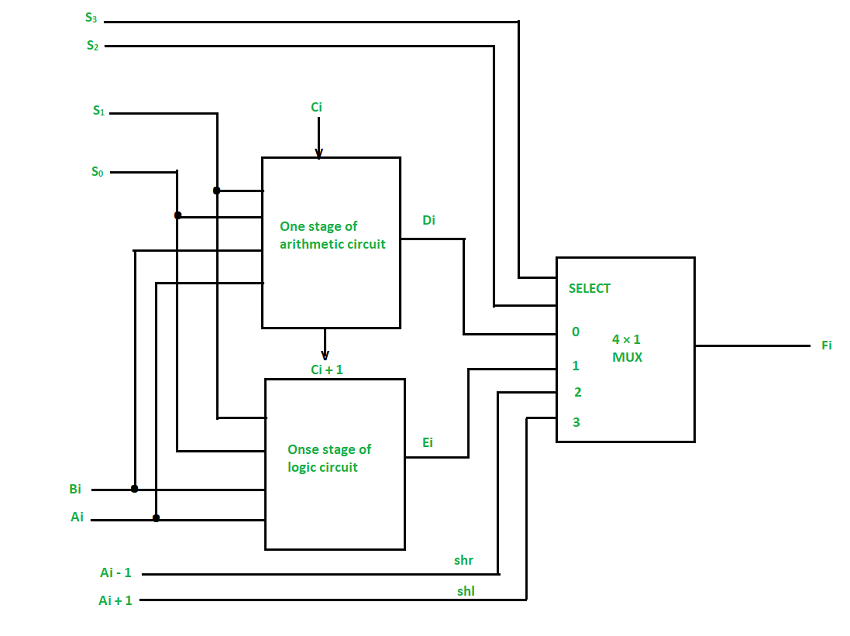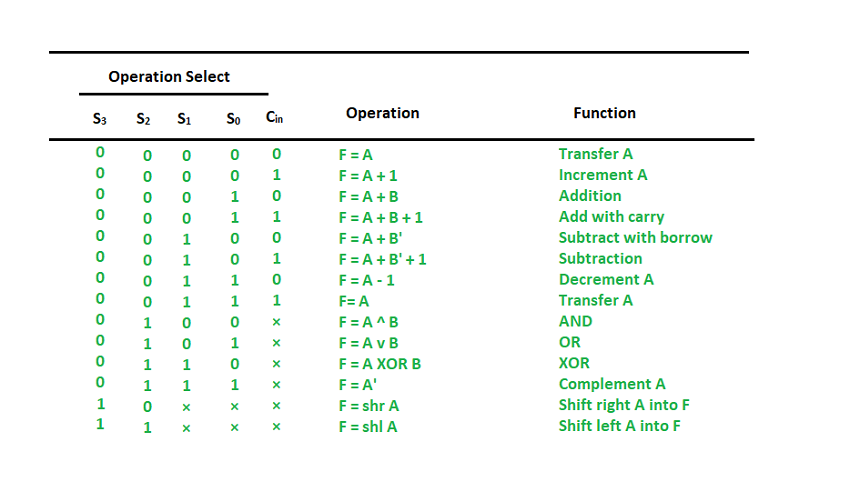Open in App
Not now

# Arithmetic Logic Shift Unit in Computer Architecture

• Difficulty Level : Easy
• Last Updated : 31 Mar, 2021

Arithmetic Logic Shift Unit (ALSU) is a member of the Arithmetic Logic Unit (ALU) in a computer system. It is a digital circuit that performs logical, arithmetic, and shift operations. Rather than having individual registers calculating the micro operations directly, the computer deploys a number of storage registers which is connected to a common operational unit known as an arithmetic logic unit or ALU.

Now, to implement the micro operation, the contents of specified registers are allocated in the inputs of the common Arithmetic Logic Unit. The Arithmetic Logic Unit performs an operation that leads as a result and gets transferred to a destination register. Arithmetic Logic Unit may be a combinatory circuit in order that the complete register transfer operation from the supply registers through the ALU and into the destination register is performed throughout one clock pulse amount. Sometimes, the shift micro operations are performed in a separate unit, but sometimes it is made as a part of full ALU.One stage of ALSU

We can combine and make one ALU with common selection variables by adding arithmetic, logic, and shift circuits. We can see the, One stage of an arithmetic logic shift unit in the diagram below. Some particular micro operations are selected through the inputs S1 and S0.

4 x 1 multiplexer at the output chooses between associate arithmetic output between Ei and a logic output in Hi. The data in the multiplexer are selected through inputs S3 and S2 and the other two data inputs to the multiplexer obtain the inputs Ai – 1 for the shr operation and Ai + 1 for the shl operation.

Note: The output carry Ci + 1 of a specified arithmetic stage must be attached to the input carry Ci of the next stage in the sequence.

The circuit whose one stage is given in the below diagram provides 8 arithmetic operations, 4 logic operations, and 2 shift operations, and Each operation is selected by the 5 variables S3, S2, S1, S0, and Cin.

The below table shows the 14 operations perform by the Arithmetic Logic Unit:

1. The first 8 are arithmetic operations which are selected by S3 S2 = 00
2. The next 4 are logic operations which are selected by S3 S2 = 01
3. The last two are shift operations which are selected by S3 S2 = 10 & 11Function table of ALSU

My Personal Notes arrow_drop_up